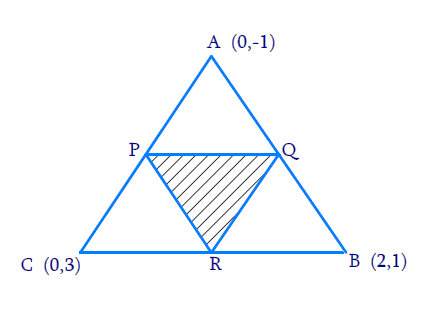# Ex.7.3 Q3 Coordinate Geometry Solution - NCERT Maths Class 10

Go back to  'Ex.7.3'

## Question

Find the area of the triangle formed by joining the mid-points of the sides of the triangle whose vertices are $$(0, -1), (2, 1)$$ and $$(0, 3).$$ Find the ratio of this area to the area of the given triangle.

## Text Solution

Reasoning:

Let ABC be any triangle whose vertices are  $$A(x_1, y_1),$$  $$B(x_2, y_2)$$ and $$C(x_3, y_3).$$

Area of a triangle

\begin{align}=\frac{1}{2} \begin{bmatrix} x_1 \left( y_2 - y_3 \right) \\ + x_2 \left( y_3 - y_1 \right) \\ + x_3 \left( y_1 - y_2 \right) \end{bmatrix} \end{align}

What is Known:

The $$x$$ and $$y$$ co-ordinates of the vertices of the triangle.

What is Unknown?

The ratio of this area to the area of the given triangle.

Solution:

From the given figure,Given,

• Let A $$(x_1, y_1) = (0, -1)$$
• Let B $$(x_2, y_2) = (2 , 1)$$
• Let C $$(x_3, y_3) = (0, 3)$$

Area of a triangle

\begin{align}=\frac{1}{2} \begin{bmatrix} x_1 \left( y_2 - y_3 \right) \\ + x_2 \left( y_3 - y_1 \right) \\ + x_3 \left( y_1 - y_2 \right) \end{bmatrix}\;\; \dots (1) \end{align}

By substituting the values of vertices, $$A, B, C$$ in $$(1)$$,

Let $$P, Q, R$$ be the mid-points of the sides of this triangle.

Coordinates of $$P, Q,$$ and $$R$$ are given by

\begin{align}{P = \left[ {\frac{{0 + 2}}{2},\frac{{ - 1 + 1}}{2}} \right] = (1,0)}\\{Q = \left[ {\frac{{0 + 0}}{2},\frac{{3 - 1}}{2}} \right] = (0,1)}\\{R = \left[ {\frac{{2 + 0}}{2},\frac{{1 + 3}}{2}} \right] = (1,2)}\end{align}

By substituting the values of Points $$P, Q, R$$

Area of $$\Delta PQR$$,

\begin{align}&= \frac{1}{2}\begin{bmatrix} (2 - 1) + 1(1 - 0) \\ + 0(0 - 2)\end{bmatrix} \\ &= \frac{1}{2}(1 + 1)\\ &= 1{\text{ Square units }}\end{align}

By substituting the values of Points $$A, B, C$$

Area of $$\Delta ABC$$,

\begin{align}&= \frac{1}{2} \begin{bmatrix} 0(1 - 3) + 2( 3 - ( - 1)) \\ + 0( - 1 - 1) \end{bmatrix} \\ &= \frac{1}{2}(8) \\ &= 4{\text{ Square units }}\end{align}

Therefore, Ratio of this area $$\Delta \, PQR$$ to the area of the triangle \begin{align}\Delta {\text{ABC = 1: 4}}\end{align}

Learn from the best math teachers and top your exams

• Live one on one classroom and doubt clearing
• Practice worksheets in and after class for conceptual clarity
• Personalized curriculum to keep up with school